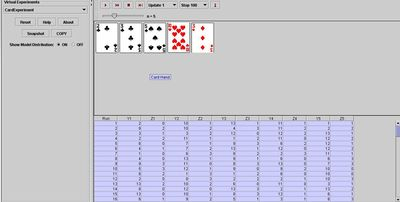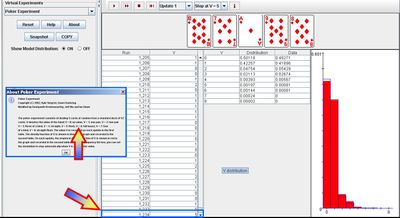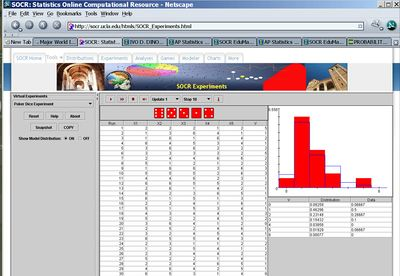# AP Statistics Curriculum 2007 Prob Count

## General Advance-Placement (AP) Statistics Curriculum - Counting Principles in Probability Theory

### Counting Principles in Probability Theory

There are many useful counting principles to compute the number of ways that certain arrangements of objects can be formed. Examples of such problems are counting combinations or permutations of objects. For a given collection of objects {$$a_i$$}, counting methods help us describe protocols for computing numbers of arrangements of these objects when taken n at a time. For instance the number of different possible orderings of a deck of n cards is $$1\times 2\times 3 \times 4 \times \cdots \times n = n!$$ (i.e., as $$n \longrightarrow \infty$$).

$$n! \approx \sqrt{2\pi n}\, \left(\frac{n}{e}\right)^{n}$$

### Permutations

Permutation is the rearrangement of objects or symbols into distinguishable sequences. Each unique ordering is called a permutation. For example, with the numbers {1, 2, 3, 4, 5, 6}, each possible ordering consists of a complete list of the numerals, without repetitions. There are 6!=720 total number of permutations of these numerals, one of which is: {6, 5, 4, 3, 2, 1}.

#### Permutations with repetitions

When the ordering of objects matters, and an object can be chosen more than once, the number of permutations is $$n^r$$, where n is the number of objects from which you can choose and r is the number of objects we can choose (repetitions allowed). For example, if you have the letters A, B, C, and D and you wish to discover the number of ways to arrange them in three letter patterns, then there are $$4^3$$ or 64 arrangements. Note that in this case:

• order matters (e.g., A-B is different from B-A, both are included as possibilities);
• an object can be chosen more than once (A-A possible).

This is because for the first slot you can choose any of the four values, for the second slot you can choose any of the four, and for the final slot you can choose any of the four letters. Multiplying them together gives the total.

#### Permutations without repetitions

When the order matters and each object can be chosen only once, then the number of permutations is $$n\times (n-1)\times (n-2) \times \cdots \times (n-r+1) = \frac{n!}{(n-r)!}$$, where n is the number of objects from which you can choose, r is the number to be chosen and $$k! = 1\times 2\times 3 \times \cdots \times k$$ is the factorial notation. For example, if we have 5 people and we choose 3 out of the 5, we have 5!/(5 − 3)! = 60 permutations.

• If n = r (meaning the number of chosen elements is equal to the number of elements to choose from; we pick all 5 people) then the number of permutation becomes $$\frac{n!}{(n-n)!} = \frac{n!}{0!} = n!$$, with 0! = 1 (by definition).
• If we have 5 people and want to arrange them all, the number of possible arrangements (permutations) will be 5! or 5 × 4 × 3 × 2 × 1 = 120 ways. The reason for this is that you can choose from 5 for the initial slot, then you are left with only 4 to choose from for the second slot etc. Multiplying them together gives the total of 120.

#### Hands-on permutation activity

This SOCR Matching Experiment demonstrates the concept of permutation. This experiment allows us to randomly permute n balls, numbered 1 to n. A match occurs whenever the ball number and the position number agree. The matches are shown in red. The number of matches N is recorded on each update. The distribution and moments of N are shown in the distribution graph and the distribution table. The parameter n can be varied with a scroll bar. Two derivations of the matching problem probabilities using permutations can be found here.### Combinations

An ordered collection of objects is a permutation. A combination is an un-ordered collection of unique objects. Given S, the set of all possible unique elements, a combination is a subset of the elements of S. The order of the elements in a combination is not important (two lists with the same elements in different orders are considered to be the same combination). Also, the elements cannot be repeated in a combination (every element appears uniquely once); i.e., we sample without replacement/repetition. Combinations are defined by the elements contained in them, thus the set {1,1,2} is the same as {2,1,1}. For example, from a 52-card deck any 5 cards can form a valid combination (a hand). The order of the cards doesn't matter and there can be no repetition of cards.

#### Combinations without repetitions

When the order does not matter and each object can be chosen only once, the number of combinations is the binomial coefficient${n\choose k} = {{n!} \over {k!(n - k)!}}$, where n is the number of objects from which you can choose and k is the number of chosen objects. For example, if you have ten numbers and wish to choose 5 you would have 10!/(5!(10 − 5)!) = 252 ways to choose. The binomial coefficient is also used to calculate the number of permutations in a lottery, as the order of the lottery numbers is irrelevant. You can use this combinations calculator to compute the combinations for $$1\leq n \leq 1,000$$.

#### Combinations with repetitions

When the order does not matter and an object can be chosen more than once, then the number of combinations is $${{(n + k - 1)!} \over {k!(n - 1)!}} = {{n + k - 1} \choose {k}} = {{n + k - 1} \choose {n - 1}}$$, where n is the number of objects from which you can choose and k is the number to be chosen. For example, if you have ten types of doughnuts (n) on a menu to choose from and you want three doughnuts (k) there are (10 + 3 − 1)! / 3!(10 − 1)! = 220 ways to choose.

To understand this formula, consider the n=10 doughnuts as 10 categories $$\{D_1, D_2, \cdots, D_{10}\}$$, and the combination of 3 doughnuts , with repetitions, as arrangements. So several examples include:

Doughnut types$\{D_1 , D_2 , D_3 , D_4 , D_5 , D_6 , D_7 , D_8 , D_9 , D_{10}\}$
Example selection$\{1 1, 0 , 1, 0, 0, 0, 0, 0, 0, 0\}$ (one repetition).
Another selection$\{0 , 0 , 1, 0, 0, 1, 0, 0, 0, 1\}$ (no repetitions).

Now let's list these arrangements as strings of 1's and 0's, where ones indicate doughnuts of the specified type, and zeros indicate spaces between doughnut categories (types): $\{1 1 0 0 1 0 0 0 0 0 0 0\}$, first arrangement of 3 doughnuts containing two doughnuts of Type 1, one of Type 3, where the nine zeros represent the nine boundaries between the 10 doughnut types. $\{0 0 1 0 1 0 1 0 0 0 0 0\}$, second arrangement of 3 doughnuts containing one doughnut of Type 3, one of Type 4, and one of Type 5.

etc.

We set an order of the categories and count how many from each category are chosen. Each combination will contain three 1’s, and nine 0's (between doughnut-group category divisions). We use 0's to track the transitions between doughnut types. Thus, associated with each event is a binary string of 1’s (doughnut types to be chosen) and 0’s (9 transitions between the 10 doughnut categories). Hence we have $${{(n + k - 1)!} \over {k!(n - 1)!}} = {{10 + 3 - 1} \choose {3}} = {{12} \choose {3}} = 220$$ combinations (with possible doughnut type repetitions).

#### Hands-on combination activity

The SOCR Card Experiment allows us to see how the number of combinations may be used to estimate probabilities of various events (in terms of card hand outcomes). Suppose we are interested in the probability that all 5 cards in a randomly drawn hand are of the same suit (flush).

• Approximation: And we can also use the Card Experiment to estimate this probability. For example, we can run the experiment 100 times. Let T be the number of 5-card hands that had all cards of the same suit (i.e., $$Z_i=Z_j$$, for all $$1 \leq i,j \leq 5$$). Then Z/100 gives a simulation-based estimate of the probability of the complex event of interest (at least one pair). Note that the values of $$Z_i$$ are clubs (0), diamonds (1), hearts (2) and spades (3). Thus, T is the number of rows, in the output table, that have all Z-columns having the same value (0, 1, 2, or 3). In one experiment of 100 runs, we observed one 5-card hand where all cards had the same suit. Therefore the approximate probability of a flush (the event that in a randomly chosen 5-card hand, all cards are of the same suit) is 1/100 = 0.01. This can be done by copying from the SOCR Card Applet all the rows of the output table (CNT-C) and pasting these data in an Excel spreadsheet. Then use the following function IF(MIN(C2, E2, G2, I2, K2)-MAX(C2, E2, G2,I2, K2)=0,1,0), to construct a new column (L) that looks at the Z (suit) columns and assigns 1 (5 matching suits, flush) or 0 (no flush) for each 5-card hand. At the end, the sum of the values in this column (=SUM(L2:L101)) will represent the frequency of occurrence of a flush in the sample (in this case one-hundred 5-card hands).• Alternative Approximation: We can also use the Poker Experiment to estimate the probability of a flush (V=5). Choose a stopping criterion of V=5 (this is flush). Then run the experiment. It will stop the first time you observe a flush. Therefore, 1/(number of experiments) is an estimate of the desired probability of observing a flush (see image below) - in this case 1/1,234.#### Poker Game Calculations

There is a variety of events that may be of interest for 5-card (poker) games (see also this section on card games. Some of these are:

• A single Pair Match: For instance, the hand with the pattern AABCD, where A, B, C and D are from the distinct kinds (denominations) of cards: aces, twos, threes, tens, jacks, queens, and kings (there are 13 denominations, and four suits, in the standard 52 card deck). The number of such hands is $${13 \choose 1}{4\choose 2}{12\choose 3}{4\choose 1}^3$$. If all hands are equally likely, the probability of a single pair is obtained by dividing this number by the total number of 5-card hands possible ($${52\choose 5}=2,598,960$$). Thus, P(1 pair only) = 0.422569.
• Two pairs: For instance, the pattern AABBC where A, B, and C are from distinct kinds. The number of such hands is $${13\choose 2}{4\choose 2}{4\choose 2}{11\choose 1}{4\choose 1}$$. And therefore, dividing by $${52\choose 5}=2,598,960$$, the P(2 pairs) = 0.047539.
• One triple: For example, the pattern AAABC where A, B, and C are from distinct kinds. The number of such hands is $${13\choose 1}{4\choose 3}{12\choose 2}{4\choose 1}^2$$. Thus, the P(1 triple) = 0.021128.
• A Full House: This includes patterns like AAABB where A and B are from distinct kinds. The number of such hands is $${13\choose 1}{4\choose 3}{12\choose 1}{4\choose 2}$$. Thus, P(Full House)=0.001441.
• Four of a kind: For instance, the pattern AAAAB where A and B are from distinct kinds. The number of such hands is $${13\choose 1}{4\choose 4}{12\choose 1}{4\choose 1}$$. The P(4-of-a-kind)=0.000240.
• A straight: Five cards in a sequence (e.g., 4,5,6,7,8), with aces allowed to be either 1 or 13 (low or high) and with the cards allowed to be of the same suit (e.g., all hearts) or from some different suits. The number of such hands is $$10*{4\choose 1}^5$$. Thus, P(A Straight)=0.003940. But if you exclude Straight-Flushes AND Royal Flushes, the number of such hands is $$10*{4\choose 1}^5 - 36 - 4 = 10200$$, the corresponding probability P(A Straight, but not a Straight or Royal Flush)=0.00392465.
• A Straight Flush: All 5 cards are from the same suit and they form a straight. The number of such hands is 4*10, and P(Straight Flush)=0.0000153908.
• A Royal Flush: This consists of the ten, jack, queen, king, and ace all in one suit. There are only 4 such hands. Thus, P(Royal Flush)=0.00000153908.### Applications

#### Number of integer solutions to linear equations

• Preview: Suppose we have n balls that we want to randomly position in r distinguishable urns, assume $$n\ge r$$. What is the number of possible arrangements of these balls?
• If the balls are distinguishable (labeled)$n^r$ possible outcomes, where empty urns are permitted. Each of the n balls can be placed in any of the r urns.
• If the balls are indistinguishable: no empty urns are allowed – select r-1 of all possible n-1 dividing points between the n balls. Empty urns are allowed.
• There are $${{n - 1} \choose {r-1}}$$ distinct positive integer-valued vectors $$\{x_1, x_2, \cdots , x_r \}$$ satisfying:

$x_1+ x_2 + \cdots + x_r = n$, where $$x_i>0$$, for $$1\leq i\leq r$$. 2) Suppose now the values are non-negative. Since there are n+r-1 possible positions for the dividing splitters (or by letting $$y_i=x_i+1$$ in the previous bullet, the right-hand-size becomes equal to n+r). There are $${{n +r - 1} \choose {r-1}}$$ distinct positive integer-valued vectors $$\{y_1, y_2, \cdots , y_r \}$$ satisfying: $y_1+ y_2 + \cdots + y_r = n$, where $$y_i \geq 0$$, for $$1\leq i\leq r$$.

• Example: An investor has $20K (n) to invest in 4 (r) potential stocks. To minimize transaction fees, each investment is in increments of$1k. In how many different ways can the money be invested?

$x_1+x_2+x_3+x_4=20$, $$x_k\ge 0$$

${{20 + 4 -1} \choose {4-1}} = 1,771.$
If not all the money needs to be invested, let x_5 be the left over money, then $$x_1+x_2+x_3+x_4+x_5=20$$:
${{24} \choose {4}} = 10,626.$